Updated by cyoumans on May 20, 2020
REPORT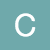cyoumans
Owner
8 items   1 followers   0 votes   63 views

# Youmans 5th Grade Gifted Math: Web Activities for Solving Problems with Decimals

Standard Covered:
MGSE5.NBT.7 Add, subtract, multiply, and divide decimals to hundredths, using concrete models or drawings and strategies based on place value, properties of operations, and/or the relationship between addition and subtraction; relate the strategy to a written method and explain the reasoning used.

2

## Solving More Decimal Word Problems | Math GoodiesThis site provides you with examples of multiplication and division word problems with decimals and how they solved them. There are some practice problems at the bottom, but they will not explain your error if you do it incorrectly. Review the example problems, but skip over the estimation ones.

2

## Key Words Used in Math Word Problems - English Study Here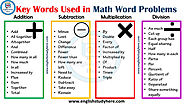Review the Key words you look for when solving multiplication and division word problems. These are the terms you will be looking for when you work to solve DECIMAL multiplication and division word problems as well. Take notes if needed.

4

## IXL | Multiply two decimals | 5th grade math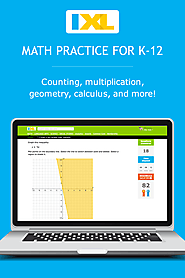Practice Time! Here is another place to practice your decimal multiplication skills. Remember it is ok if you get an incorrect answer. The site will guide you through the correct way to solve it if that happens.

6

## Multiplying decimals example (video) | Khan Academy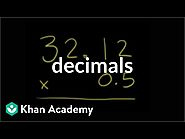This link will take you to a video that shows how to multiply decimals. On the left hand side of the page, you will see links to other videos and practice for both multiplying and dividing decimals. You may find these videos more helpful than the others posted or vice versa. You choose which works best for you.

10

## Dividing Decimals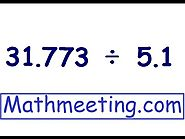This video shows how to divide decimals. Watch each step and take notes if needed to ensure you understand. The method is the same no matter how many digits are in front of the decimal. NOTE: This video will pop up at the bottom of your current page. Scroll down and be sure your sound is on to watch the video.

10

## Multiplying Decimals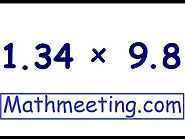This video shows how to multiply decimals. Watch each step and take notes if needed to ensure you understand. Although it only shows one digit decimal numbers, the method is the same no matter how many digits are in front of the decimal. NOTE: This video will pop up at the bottom of your current page. Scroll down and be sure your sound is on to watch the video.

7

## Dividing DecimalsThis page has a visual explanation of dividing decimals, without a video. There is also an animation link you can view. At the bottom you will see a box that says "your turn". Click on Question 1 to begin and test out your knowledge. It's ok if you get it wrong. This is practice and this site will explain the problem if you choose the incorrect answer.

8

## Multiplying DecimalsThis page has a visual explanation of multiplying decimals, without a video. At the bottom you will see a box that says "your turn". Click on Question 1 to begin and test out your knowledge. It's ok if you get it wrong. This is practice and this site will explain the problem if you choose the incorrect answer.